Updating search results...

# 5 Results

View
Selected filters:
• length-model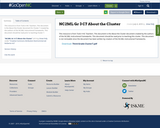Only Sharing Permitted
CC BY-NC-ND
Rating
0.0 stars

This resource is from Tools 4 NC Teachers. This document is the About the Cluster document for Cluster 7 created by the authors of the NC2ML Instructional Frameworks. This document should be read prior to teaching Cluster 7.

Subject:
Mathematics
Material Type:
Reference Material
07/28/2020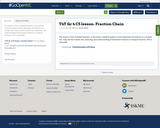Conditional Remix & Share Permitted
CC BY-NC-SA
Rating
0.0 stars

This lesson is from Tools4NCTeachers. In this lesson, students explore correct placement of fractions on a number line. They use the number line, reasoning, and understanding of benchmark fractions to compare fractions. This is remixable.

Subject:
Mathematics
Material Type:
Activity/Lab
11/06/2019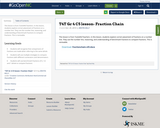Conditional Remix & Share Permitted
CC BY-NC-SA
Rating
0.0 stars

This lesson is from Tools4NCTeachers. In this lesson, students explore correct placement of fractions on a number line. They use the number line, reasoning, and understanding of benchmark fractions to compare fractions. This is remixable.

Subject:
Mathematics
Material Type:
Activity/Lab
11/06/2019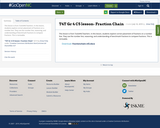Conditional Remix & Share Permitted
CC BY-NC-SA
Rating
0.0 stars

This lesson is from Tools4NCTeachers. In this lesson, students explore correct placement of fractions on a number line. They use the number line, reasoning, and understanding of benchmark fractions to compare fractions. This is remixable.

Subject:
Mathematics
Material Type:
Activity/Lab
11/06/2019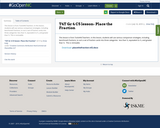Conditional Remix & Share Permitted
CC BY-NC-SA
Rating
0.0 stars

This lesson is from Tools4NCTeachers. In this lesson, students will use various comparison strategies, including benchmark fractions, to sort a set of fraction cards into three categories: less than _, equivalent to _, and greater than _. This is remixable.

Subject:
Mathematics
Material Type:
Activity/Lab
Lesson Plan